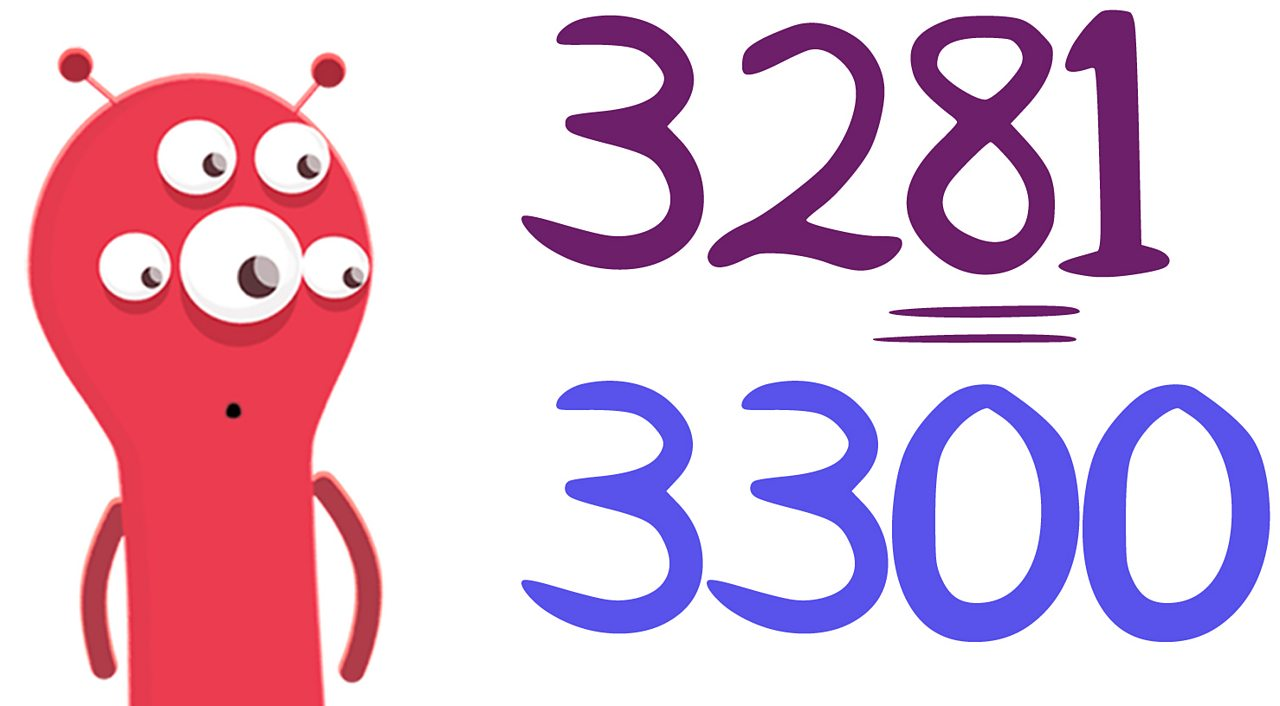# How to round numbers

## Rounding to the nearest 10

To round a number to the nearest 10, look at the units digit. If the units digit is 5 or more, round up. If the units digit is 4 or less, round down.

• The last digit in 356 is 6. So we round it up to 360.
• The last digit of 352 is 2. So we round it down to 350.
• 475 ends in a 5. We always round a 5 up. So 475 rounds up to 480.

## Rounding to the nearest 100

To round a number to the nearest 100, look at the tens digit. If the tens digit is 5 or more, round up. If the tens digit is 4 or less, round down.

• The tens digit in 3281 is 8. So we round it up to 3300.
• The tens digit of 3216 is 1. So we round it down to 3200When rounding to the nearest 100, 3281 becomes 3300.

## Rounding to the nearest 1000

To round a number to the nearest 1000, look at the hundreds digit. If the hundreds digit is 5 or more, round up. If the hundreds digit is 4 or less, round down.

• The hundreds digit in 4559 is 5. So we round it up to 5000.
• The hundreds digit of 4295 is 2. So we round it down to 4000.